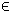## 27QCP Problem

The general formulation in TOMLAB for a quadratic complementarity problem is:

 min x
f(x) =
1
2
xT F x + cT x

s/t
 xL ≤ x ≤ xU bL ≤ A x ≤ bU
(31)
where c, x, xL, xURn, FRn × n, ARm1 × n, and bL,bURm1. Equality constraints are defined by setting the lower bound equal to the upper bound, i.e. for constraint i: bL(i) = bU(i). Fixed variables are handled the same way.
The complementarity conditions can be any combination of decision variables and linear constraints:
• x(i) ⊥ x(j)
• x(i) ⊥ A(j,:) x
• A(i,:) xA(j,:) x
The following file defines a problem in TOMLAB.

File: tomlab/quickguide/qcpQG.m

Open the file for viewing, and execute qcpQG in Matlab.
```% qcpQG is a small quadratic complementary quick guide example
%
% minimize  f = (x(1)-5)^2 + (2*x(2)+1)^2
%
% subject to
%
%    2*(x(2)-1) - 1.5*x(1) + x(3) - 0.5*x(4) + x(5) == 0   % c(1)
%     3*x(1) - x(2) - 3     >= 0                           % c(2)
%      -x(1) + 0.5*x(2) + 4 >= 0                           % c(3)
%      -x(1) -     x(2) + 7 >= 0                           % c(4)
%
%    x(1:5) >= 0
%
%    Complementarity conditions:
%
%    x(3) _|_ c(2)
%    x(4) _|_ c(3)
%    x(5) _|_ c(4)

%  If we omit the constant term 27 from f(x), we can write f = x'*F*x + c'*x:

F      = zeros(5,5);
F(1,1) = 1;
F(2,2) = 4;
c      = [-10,4,0,0,0]';

% Linear constraints, by moving constant terms in c1-c4 to the right hand
% side:
A = [...
-1.5   2.0  1.0  -0.5  1.0 ; ...
3.0  -1.0  0     0    0   ; ...
-1.0   0.5  0     0    0   ; ...
-1.0  -1.0  0     0    0 ];

b_L = [ 2 , 3   , -4  , -7 ]';
b_U = [ 2 , inf , inf , inf]';

% Lower and upper bounds
x_L =    zeros(5,1);
x_U = inf*ones(5,1);
x_0 = [];

Name = 'JF-BARD-1998-QP';

% Complementarity pairs:
mpec = [ ...
3,0, 2,0, 0,0 ; ...
4,0, 3,0, 0,0 ; ...
5,0, 4,0, 0,0 ]

% Assign a TOMLAB 'qp' problem:
Prob = qcpAssign(F, c, A, b_L, b_U, x_L, x_U, x_0, mpec, Name);

% Three slacks have been added to the problem, easy to see by looking at the
% new linear constraint matrix:
A = Prob.A

% and the original linear constraint matrix:
A_orig = Prob.orgProb.A

% Enable some crossover iterations, to "polish" the solution a bit in
% case KNITRO chooses an interior point algorithm:

Prob.KNITRO.options.MAXCROSSIT = 100;
Prob.PriLevOpt = 2;

% Solve the QP (with MPEC pairs) using KNITRO:
Result = tomRun('knitro',Prob,2);

x = Result.x_k

% Values of slacks that were added by BuildMPEC
s = x(6:8)

% Original A * original variables, subtract the constants in the
% constraints to get the result on the c(x) >= 0 form

ax = A(:,1:5) * x(1:5) - b_L

% These are now complementary:
ax(2:4), x(3:5)

% Should be zero, or very close to zero:
ax(2:4)'*x(3:5)```